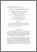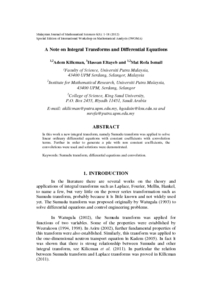Simple Search:

# A note on integral transforms and differential equations

## Citation

Kilicman, Adem and Eltayeb, Hassan and Ismail, Mat Rofa (2012) A note on integral transforms and differential equations. Malaysian Journal of Mathematical Sciences, 6 (S). pp. 1-18. ISSN 1823-8343; ESSN: 2289-750X

## Abstract / Synopsis

In this work a new integral transform, namely Sumudu transform was applied to solve linear ordinary differential equations with constant coefficients with convolution terms. Further in order to generate a pde with non constant coefficients, the convolutions were used and solutions were demonstrated.Preview
PDF
39035.pdfView Item